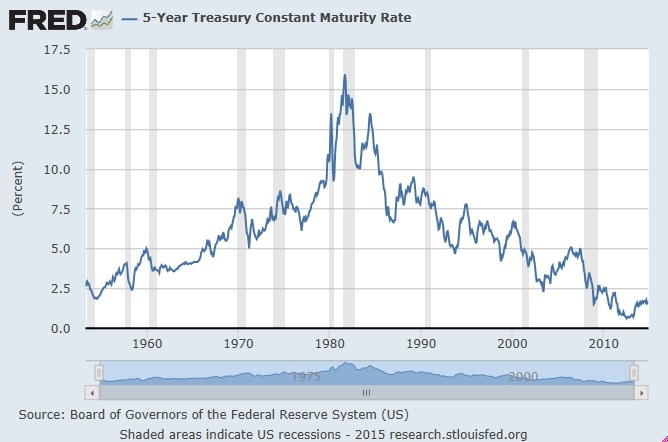# TSP F Fund’s Last Hurrah

The TSP F Fund attempts to match the performance of the Barclays Capital U.S. Aggregate Bond Index representing the U.S. bond market.  While the credit risk is low, the primary risk to the F fund comes in the form of rising interest rates.  Since its inception in 1988, the TSP F fund has had a significant tail wind of a downward trend in interest rates.  This tail wind has added approximately 1.25% to the F funds annualized return of 6.87% as reported at the end of 2013.  The remaining 5.22% comes from the average yield since inception.  With a yield today of around 2%, the return since inception is very misleading going forward.

The TSP F fund and its tracked index do not post what part of its return is from yield and what part is from capital gains or losses.  So let’s use the 5-Year Treasury Constant Maturity Rate (TCMR) as a proxy to understand how much interest rates have declined since the fund’s inception in 1988.  Then we can approximate how much of the TSP F fund’s return was from price appreciation and how much from yield.The TCMR’s 1988 average yield was 8.67%, but interest rates climbed 1% during the course of that year.  We will use the simple formula found on Barclay’s fact sheet that tells us a 1% rise in interest rates will result in approximately a 5% capital loss.  If you subtract 5% due to the rise in interest rates from the starting interest rate of 8.67% you get a 3.67% return.  TSP reported a 3.63% return for the F fund that first year.  The second year interest rates reversed and declined 1% resulting in a 5% price gain.   If you add 5% to the second years average 8.64% yield you obtain 13.64%.  TSP reported a 13.89% return in 1989.

The starting yield in 1988 was 8.2% and by 2014 the yield is down to 1.7%.   We can calculate that the 6.5% drop in interest rates added roughly 32.5% in capital appreciation since 1988.  The TSP F fund yield will lag somewhat, but our numbers are designed to make a point.  Since we are bouncing off historically low interest rates, the tail wind will become a headwind or at the very least we lose our tail wind and are stuck with today’s measly yield.

If a 1% rise in interest rates wipes 5% from the price of the TSP F funds underlying securities, then a half of a percent rise in interest rates will zero out the meager approximate 2% yield we are receiving today.  In 2013 this occurred.  Looking at our proxy yield, 2013 saw a .84% increase in interest rates.  If we multiplied this by 5 means then a 4.2% price drop occurred offset by the current yield.  The TSP F fund posted a -1.68% return which implies a 2.5% average yield in 2013.  This is about right.

With a yield of around 2%, the TSP F fund’s return is very dependent on the movement of interest rates.  Remember, a half percent jump in interest rates will more than wipe out the entire years yield.  Since we are presently in a financial environment where interest rates are at historical lows, can they continue down?  Possibly if we become a basket case like Japan.  But at 1.64% on the TCMR, if yields go to zero you will only squeeze out 8.2% more in price appreciation and with no future yield.  If interest rates stay steady then expect only a 2% return at best.

Better than the G fund you say?  But the TSP G fund cannot lose money and only comes with inflation risk.  The TSP F fund can lose money and if the Federal Reserve was to ever normalize interest rates, and then conservatively expect a 10% capital loss.

This is of course is a far cry from what you can lose in the equity markets, but if you are weighing the difference between the TSP F fund and G fund during periods of financial stress, it helps to understand how the risks can play out.  And I believe 2015 will be a year of global financial stress that started at the periphery in 2014.

The Federal Reserve plans to raise interest rates at a slow pace, so consider if they “normalize” rates by only .75% a year for three years the TSP F fund’s return for the next three years will be zero.  If they raise rates faster, you will lose money on the front end.  A good plan would be to sit in the G fund when out of equities and wait until interest rates stabilize at a higher level.

Invest smart.

Categories: Perspectives, TSP Allocation Strategy, TSP Charts

Tags: , ,